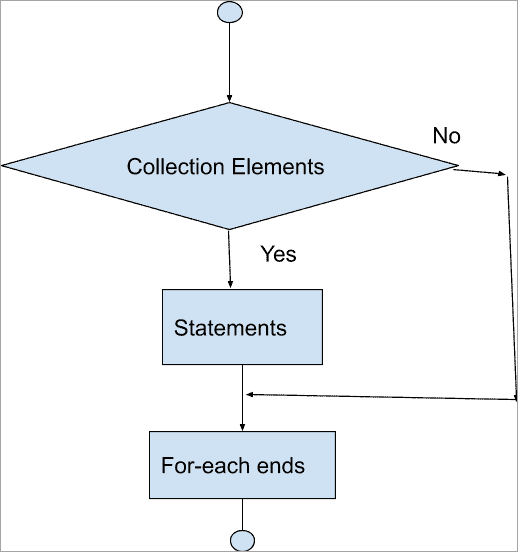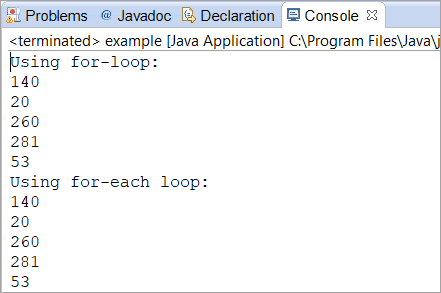# Java For Loop Tutorial With Program Examples

This tutorial will explain the concept of Java For Loop along with its syntax, description, flowchart, and programming examples:

In this tutorial, we will discuss the “for-loop” in Java. We will explore each and every aspect of the looping concept along with the way of using it.

This tutorial will be covered with enough programming examples that will let you understand various application areas of the Java for-loop. Some frequently-asked questions will also be a part of the given topic so that you will be well aware of the important questions related to the Java for-loop.

=> Check ALL Java Tutorials Here.## Java For Loop

The loop statement is an integral part of every programming language. Looping helps you to iterate each and every element based on the user-specified condition. Java is a no exception language and “for-loop” is one of the most common loops that you will see in any programming language.

Syntax:

`for (initialization; condition; iteration) statement;`

First of all, the loop control variable is initialized to its initial value. This is followed by the condition which is a boolean expression that returns either true or false. This condition is used to test the loop control variable.

If the condition holds true, then the for-loop continues its iteration otherwise it terminates.### Printing The First Ten Numbers

Given below is a simple example of Java for-loop. Here, we have printed the first ten numbers with the help of “for-loop”.

First of all, we have initialized a variable ‘i’ with the value as 1. Then we have specified a condition where “i” should be less than or equal to 10” and then we have incremented the loop by 1. As long as the value of ‘i’ is “less than or equal to 10”, then the value of ‘i’ will be printed after every iteration.

The moment its value becomes 11, then the specified condition won’t match and the loop will be terminated.

```import java.util.Scanner;

public class example {

public static void main(String[] args) {
/*
* Printing the first 10 numbers with
* the help of for-loop
*/
System.out.println("First ten numbers are: ");
for (int i=1; i <=10; i++){
System.out.println(i);
}

}

}
```

Output:### Reverse A String

In the below example, we have taken input String through the console and tried to print each of the characters in reverse order using a for-loop.

```import java.util.Scanner;

public class example {

public static void main(String[] args) {

String original, reverse = "";
System.out.println("Enter the string to be reversed");

/*
* Used Scanner class to input the String through Console
*/

Scanner in = new Scanner(System.in);
original = in.nextLine();

/*
* Using for loop, iterated through the characters
* in reverse order, decrementing the loop by -1
* and concatenating the reversed String
* using an inbuilt method charAt()
*/

int length = original.length();
for(int i=length-1; i>=0; i--) {
reverse = reverse + original.charAt(i);
}
System.out.println(reverse);
}

}
```

Output:

## Java For Each Loop

This is another form of a for-loop that is mostly used to traverse or navigate through the elements/items of a collection such as a map or arraylist. This is supported by JDK-5 and above. It is also known as enhanced for loop.

Syntax:

```for (data-type obj: array)
{
obj statement;
}
```

#### Iterating Arraylist Using A For-Each Loop

In this program, we have inserted three elements into an arraylist.

Then, we have iterated the elements of the arraylist using for-each and a for-loop as well. In the for-each loop, we have created an object called obj for the ArrayList called list and then printed the object.

In the for-loop, we have put the condition where the iterator “i” is set to 0, then it is incremented by 1 until the ArrayList limit or size is reached. Finally, we have printed each element using the get(index) method for each iteration of For Loop.

You can see that there is no difference in the output of a for-loop and for-each loop.

```import java.util.*;

public class example {
public static void main(String[] args) {
ArrayList list = new ArrayList();

// Adding elements into the arraylist

// Iterating the arraylist through the for-each loop

System.out.println("Foreach Loop:");
for(Object obj : list) {
System.out.println(obj);
}
System.out.println();

// Iterating the arraylist through for-loop

System.out.println("For Loop:");
for(int i=0; i < list.size(); i++) {
System.out.println(list.get(i));
}
}
}
```

Output:### Finding Summation Using Enhanced For-Loop

Now we are going to find the summation of the first 10 natural numbers using a for-each loop or an enhanced for loop. Here, we have declared an obj variable of type integer and after each iteration, the sum variable will have the added value of the numbers.

Finally, we have printed the sum variable to get the summation of the first 10 natural numbers.

```import java.util.*;

public class example {
public static void main(String[] args) {
int arr[] = {1,2,3,4,5,6,7,8,9,10};
int sum = 0;

/*
*  Using for-each loop to add each number and
*  Store it in the sum variable
*/

for (int obj: arr){
sum = sum + obj;
}
System.out.println("The total of first 10 natural number:" +sum);
}
}
```

Output:### Java For-Loop Array

In this section, we will learn about the different ways of iterating through an array.

Previously, we demonstrated how to iterate arraylist using for-loop or an enhanced for-loop. Now, we will iterate through an array using a for-loop and for-each loop.

In the below programming example, we have initialized an array of size = 5 with five different values and tried to iterate the array using a for-loop and a for-each loop. You can see that there is no difference in the way in which these elements are displayed by using both the loops.

```import java.util.*;

public class example {
public static void main(String[] args) {
int arr[] = new int;

//Initializing the array with five values as size is 5
arr = 140;
arr = 20;
arr = 260;
arr = 281;
arr = 53;

//Printing the elements using for loop
System.out.println("Using for-loop:");
for(int i=0; i < arr.length; i++) {
System.out.println(arr[i]);
}

//Printing the elements using for-each loop
System.out.println("Using for-each loop:");
for(int obj: arr){
System.out.println(obj);
}

}

}
```

Output:

#Q #1) How do you repeat a loop in Java??

Answer: In java, we repeat a loop using a counter variable. Most commonly, a counter variable can be i, j, or count. It totally depends on the programmer as what variable to choose.

In the below example, we have repeated a loop 5 times and then printed the “*”. This is also known as the pyramid program. The loop will be repeated unless the value of “i” and “j” becomes equal to 5.

```public class example {

public static void main(String[] args) {

for(int i=0; i < 5; i++) {
for(int j=0; j <= i; j++) {
System.out.print("*");
}
System.out.println();
}

}

}
```

Output:Q #2) How to use for-loop for a String in Java?

Answer: Given below is the program where we have used for-loop for a String variable. Here, we have initialized a for-loop with two counters to compare if the character at the “i” index and (i+1) index is equal or not. It will print the character of the (i+1) index if they are equal.

```public class example {

public static void main(String[] args) {

String str = new String("Microsofft");
int count = 0;
char[] chars = str.toCharArray();
System.out.println("Duplicate characters are:");

/*
*  initialized a for-loop with two counters
*  to compare if character at i index and i+1 index
*  are equal or not. It will print the characters
*  if they are equal.
*/

for (int i=0; i < str.length();i++) {
for(int j=i+1; j < str.length();j++) {
if (chars[i] == chars[j]) {
System.out.println(chars[j]);
count++;
break;
}

}
}
}

}
```

Output:Q #3) How to print something once in a for-loop Java?

Answer: In the below program, the value of “i” will be printed only once as we have specified the condition accordingly.

```public class example {

public static void main(String[] args) {

for (int i=0; i < 1; i++){
System.out.println("The value is: " +i);
}

}

}
```

Output:Q #4) How to come out of for-loop in Java?

Answer: This is the most basic question of a for-loop. In the Java for-loop, as soon as the condition does not satisfy, it will automatically throw you out of the loop.

However, you can also explicitly use a break statement in Java if in case you want to come out of the loop.

With Break:

```public class example {

public static void main(String[] args) {

for (int i=0; i < 2; i++){
System.out.println("The value is: " +i);
break;
}

}

}
```

Output:Without Break:

```public class example {

public static void main(String[] args) {

for (int i=0; i < 2; i++){
System.out.println("The value is: " +i);

}

}

}
```

Output:Q #5) How to get a value from for-loop in Java?

Answer: You can get a value from the for-loop by printing the value of the counter variable (such as i, j, or count).

Q #6) How to use the for each loop in Java?

Answer: You can go through the “Java for-each loop” section of this tutorial. However, we have listed a simple example of a Java for-each loop or Java enhanced for-loop below.

```import java.util.HashMap;

public class example {

public static void main(String[] args) {

int[] arr = {2,3,9,5};

/*
* Enhanced for-loop or for-each loop
* begins here
*/

for (int obj: arr){
System.out.println(obj);
}
}
}
```

Output:## Conclusion

In this tutorial, we have explained the concept of Java for-loop along with its syntax, description, flowchart, and programming examples. The other variations of Java for-loop are also described in detail with the flowchart, description, syntax, and programming examples wherever required.

Suggested reading =>> While Loop in Java

The examples that are listed in this tutorial are very important as they are asked during the Java interviews too. We have listed a few FAQs that are again very important as they will let you understand the topic in detail.